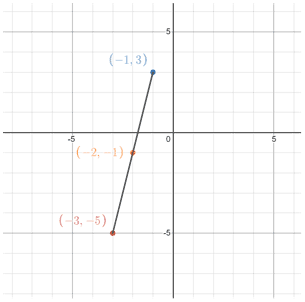# Midpoint formula

The midpoint formula is a formula used to find the halfway point between two coordinates on a graph. Given a line segment with endpoints A and B, the midpoint is the point located exactly between A and B, meaning that it is the same distance from A and B, as in the figure below.

The midpoint formula can be used when two points on a graph in the coordinate plane are known. Given two points (x1, y1) and (x2, y2), their midpoint M is:Notice that the midpoint formula involves taking an average of the x- and y-values of two coordinates. This can make it easier to remember the midpoint formula. The average of two numbers is just the sum of the two numbers divided by 2. The midpoint of a line segment in the coordinate plane is located at the vertical and horizontal halfway points between two points, which is equivalent to the average between the x-coordinates, and the average between the y-coordinates of the line segment. Remembering this should make it relatively easy to recall the midpoint formula whenever needed.

Example

 (x, y) (x1, y1) (-3, 5) (-1, 3)Midpoint at (-2, -1)